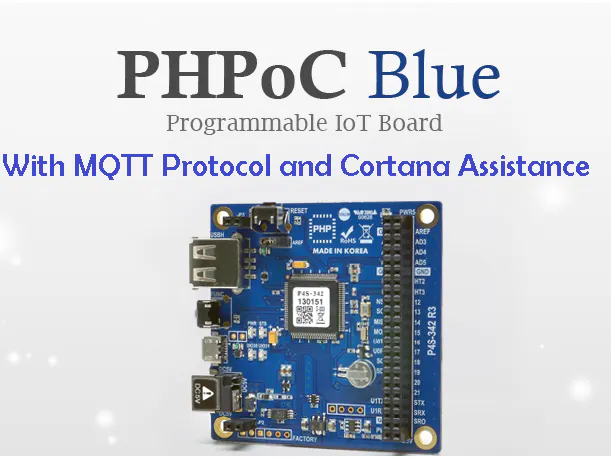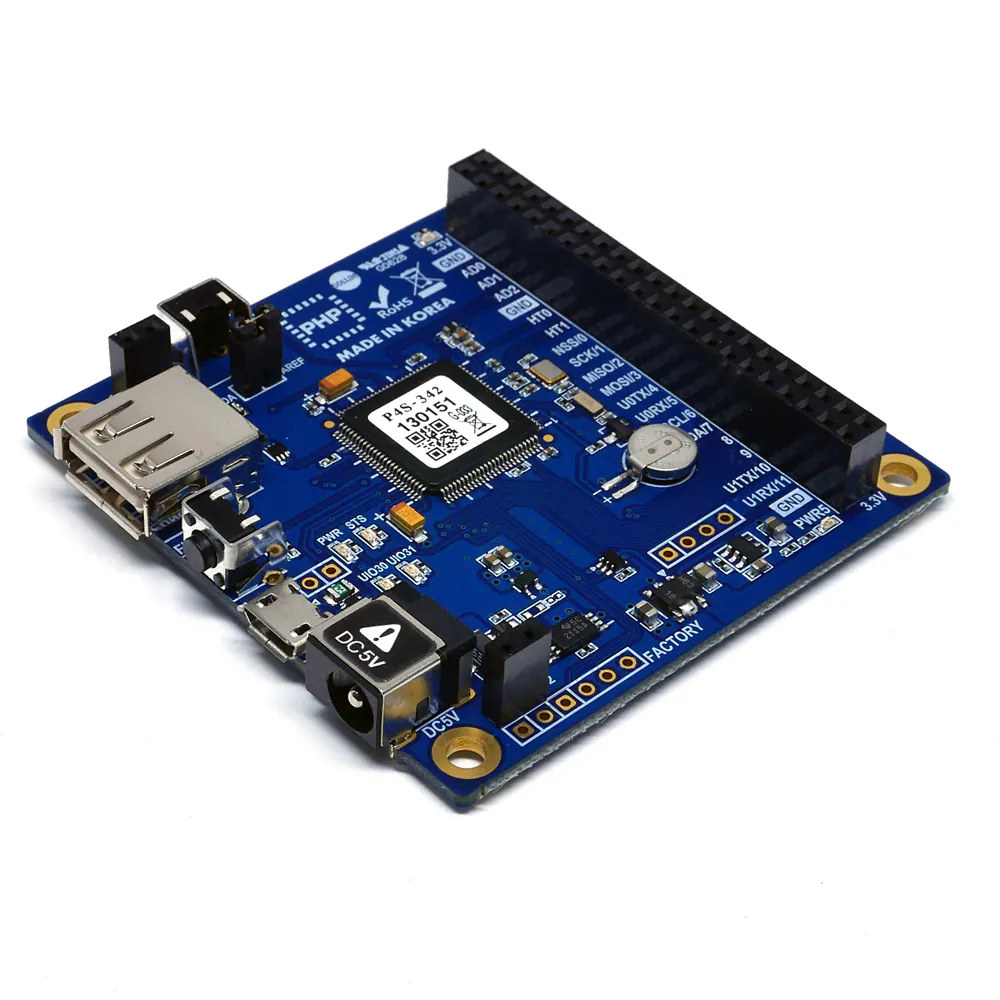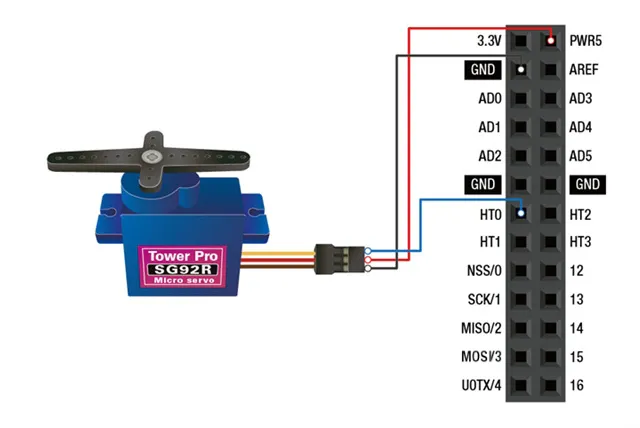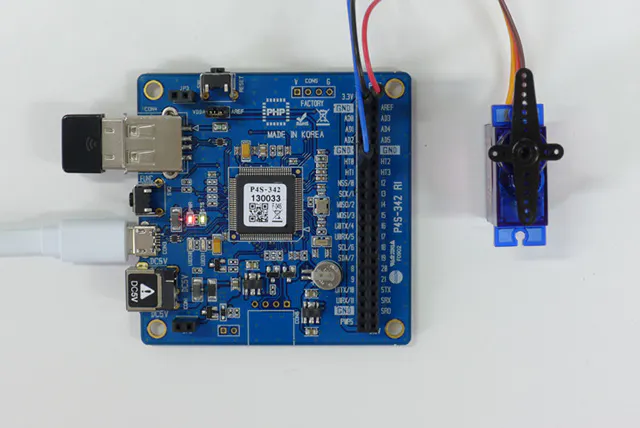Published

# PHPoc Blue to Control Door Knob by MQTT Protocol and Cortana

Programming a PHPoc Blue board to control a servo motor to rotate the knob of a door using MQTT Protocol through voice command.

IntermediateShowcase (no instructions)10 hours1,340## Things used in this project

### Hardware components

 Servos (Tower Pro MG996R) Servo motor has to be with more 250 torque
×1PHPoC Blue
×1

### Software apps and online servicesMicrosoft Visual Studio 2015PHPoC Debugger

## Schematics

### Connect the Servo motor with Board

simple diagram to connect the servo motor with board### the motor Connected

a photo after we connect the servo motor with PHPoc Blue Board## Code

### task0 file the main file

PHP
it used to program the board
```<?php
//edited By ibrahim al nasser 2017
// this code originally from Phpoc website i edit this
// to fit the requirements an also correct some errors
// from practical point of view
if(_SERVER("REQUEST_METHOD"))
exit; // avoid php execution via http request

include_once "/lib/sn_dns.php";
include_once "/lib/vn_mqtt.php";
include_once "/lib/sd_340.php";

define("PWM_PERIOD", 20000); // 20000us (20ms)
define("WIDTH_MIN", 600);
define("WIDTH_MAX", 2450);
uio_setup(0, 30, "out high");
uio_setup(0, 31, "out high");

\$flags = 0;
\$angle = 0;

if(!\$flags)
{
ht_pwm_setup(0, (WIDTH_MIN + WIDTH_MAX) / 2, PWM_PERIOD, "us");

\$flags |= 0x00000001; // set init flag
um_write(0, 0, int2bin(\$flags, 4)); // write flags (offset 0, 32bit integer)

\$angle = 90;
um_write(0, 4, int2bin(\$angle, 4)); // write angle (offset 4, 32bit integer)
}

//\$host_name = "test.mosquitto.org";
//\$host_name = "iot.eclipse.org";
\$host_name = "broker.hivemq.com";
//\$host_name = "broker.mqttdashboard.com";
//\$host_name = "[192.168.0.3]";
\$port = 1883;

mqtt_setup(0, "PHPoC-MQTT Sub Example",  \$host_name, \$port);

/*
\$will_qos = 2;
\$will_retain = true;
\$will_topic = "disaster/tsunami";
\$will_message = "Goodbye forever");
\$will = array(\$will_qos, \$will_retain, \$will_topic, \$will_message);
*/
\$will = "";

\$out_topics = array(array("room/open", 1), array("room/close", 2));

\$in_topic = "";
\$in_content = "";
\$is_retain = 0;

while(1)
{
while(mqtt_state() == MQTT_CONNECTED)
{
if(mqtt_subscribe(\$out_topics))
break;
}
if(mqtt_state() == MQTT_DISCONNECTED)
while(mqtt_reconnect() == false);
uio_out(0, 31, LOW);
if(mqtt_loop(\$in_topic, \$in_content, \$is_retain))
{
//TODO , procees the received publish packet here
if(\$is_retain == 1)
//	echo "<<a stale message\r\n";
uio_out(0, 30, LOW);
//	echo "<<topic:\$in_topic\r\n";
echo "<<content: \$in_content\r\n";
if(\$in_content=="openning"){
uio_out(0, 30, HIGH);

\$delta = -180; // clock wise

if(\$delta)
{

\$angle += \$delta;

if(\$angle > 180)
\$angle = 180;

if(\$angle < 0)
\$angle = 0;

um_write(0, 4, int2bin(\$angle, 4)); // write angle (offset 4, 32bit integer)

\$width = WIDTH_MIN + (int)round(\$angle / 180.0 * (WIDTH_MAX - WIDTH_MIN));

ht_pwm_width(0, \$width, PWM_PERIOD);

}
}
else{
if(\$in_content=="closing"){

\$delta = 180; // clock wise
if(\$delta)
{

\$angle += \$delta;

if(\$angle > 180)
\$angle = 180;

if(\$angle < 0)
\$angle = 0;

um_write(0, 4, int2bin(\$angle, 4)); // write angle (offset 4, 32bit integer)

\$width = WIDTH_MIN + (int)round(\$angle / 180.0 * (WIDTH_MAX - WIDTH_MIN));

ht_pwm_width(0, \$width, PWM_PERIOD);
}

}}

}
}
//mqtt_unsubscribe("sensors/temperature");

mqtt_disconnect();

?>
```

### phpoc.ini

PHP
to extend the cach memory in the board
```tcp0_txbuf_size = 4096 ; SSL/TCP send buffer
tcp0_rxbuf_size = 4096 ; SSL/TCP send buffer
ssl0_rxbuf_size = 4096
```

### init.php file

PHP
the initial file for the Phpoc Blue board
```<?php
?>
```

### Costum Cortana Commands Project

it used to program a specific commands through cortana

## Credits

### Ibrahim alnasser

0 projects • 1 follower
a Master Student in Embedded Systems Program , in TU Chemnitz